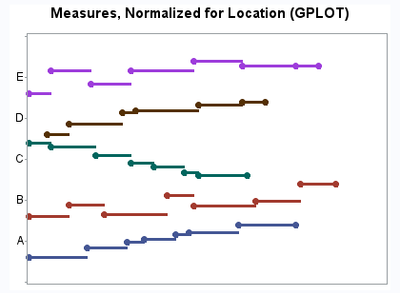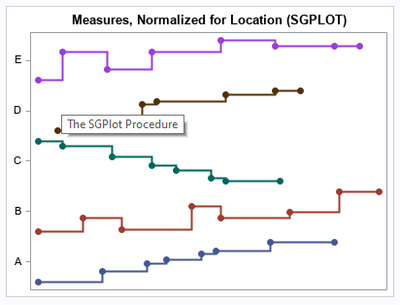## Is there an option for hiding the vertical part of the lines in a SGPLOT STEP plot ?

How can I use SGPLOT to produce a STEP plot that has the same look as a GPLOT with SYMBOL I=STEPL.

Data generator and z-normalize

Spoiler
```data have(keep=location time measure intensity);
call streaminit(202005);
location = 'A'; time=0;
do k = 1 to 7;
measure = 10 + time; intensity=1+mod(k,4); output;
time + 1+rand('integer',1,12)/2;
end;  output;

location = 'B'; time=0;
do k = k to k+6;
measure = 20 + rand('uniform'); intensity=1+mod(k,4); output;
time + 1+rand('integer',1,12)/2;
end;  output;

location = 'C'; time=0;
do k = k to k+6;
measure = 30 - time; intensity=1+mod(k,4); output;
time + 1+rand('integer',1,12)/2;
end;  output;

location = 'D'; time=0;
do k = k to k+6;
measure = 6.5 + log(time*2); intensity=1+mod(k,4); output;
time + 1+rand('integer',1,12)/2;
end;  output;

location = 'E'; time=0;
do k = k to k+6;
measure = mod(time-1,3); intensity=1+mod(k,4); output;
time + 1+rand('integer',1,12)/2;
end;  output;
run;

proc sql;
create table locations as select distinct location from have;

data location_ids;
set locations;
location_id + 1;
retain fmtname 'locid';
run;

proc format cntlin=location_ids(rename=(location_id=start location=label));
run;

proc sql;
* normalize measure by location to z in [-0.4 to 0.4] about location_id;
create table have_z as
select
have.location
, location_ids.location_id
, have.time
, have.measure
, (measure-min(measure)) / (max(measure) - min(measure)) * 0.8 - 0.4 as z_normal
, mean(location_id) + calculated z_normal as z_level format=locid.
, have.intensity
from have
join location_ids on have.location = location_ids.location
group by location_id
order by location_id, time
;

ods html file='step.html';
ods graphics / width=400px;```

GPLOT (no vertical part)

```goptions xpixels=400 ypixels=300;
symbol1 i=stepL v=dot width=2 height=2;
axis1 label=none minor=none order=0 to 6 value=('' 'A' 'B' 'C' 'D' 'E' '');
axis2 label=none minor=none major=none value=none;

proc gplot data=have_z;
title h=11pt "Measures, Normalized for Location (GPLOT)";
plot z_level * time = location_id / nolegend vaxis=axis1 haxis=axis2;
run;```SGPLOT (how to remove vertical part ?)

```proc sgplot data=have_z noautolegend;
title h=11pt "Measures, Normalized for Location (SGPLOT)";
step   x=time y=z_level / group=location_id lineattrs=(thickness=2px) markers markerattrs=(symbol=circlefilled size=8px);
xaxis integer display=none;
yaxis integer display=(nolabel);
run;```1 ACCEPTED SOLUTION

Accepted SolutionsJay54
Meteorite | Level 14

## Re: Is there an option for hiding the vertical part of the lines in a SGPLOT STEP plot ?

The GTL syntax that SGPLOT produces does support the JOIN=false option to achieve the visual you want.  SGPLOT may not implement this option.  One way to do this to run your SGPLOT code with TMPLOUT="filename" option.  The generated GTL code will be saved in filename.  Then add the JOIN option to the generated STEPPLOT statement and run the GTL program.Jay54